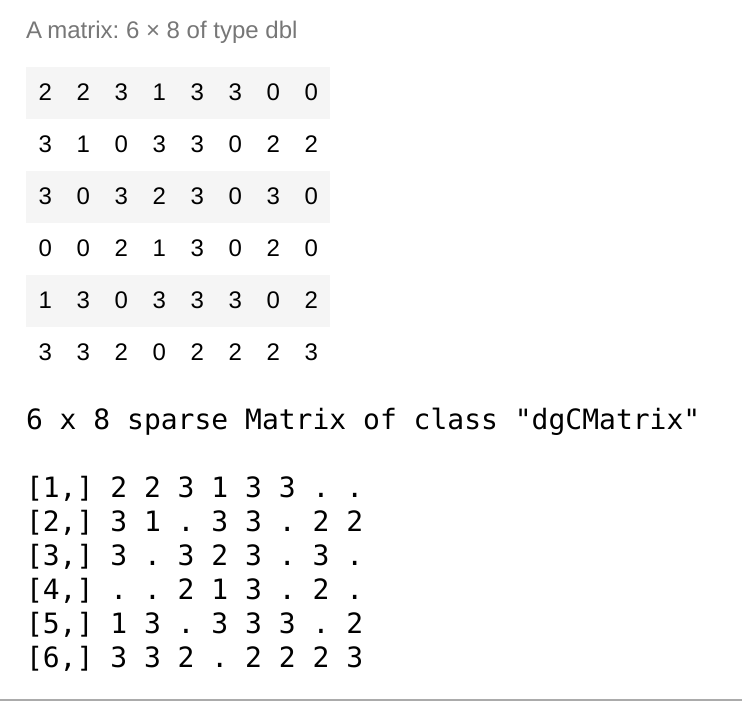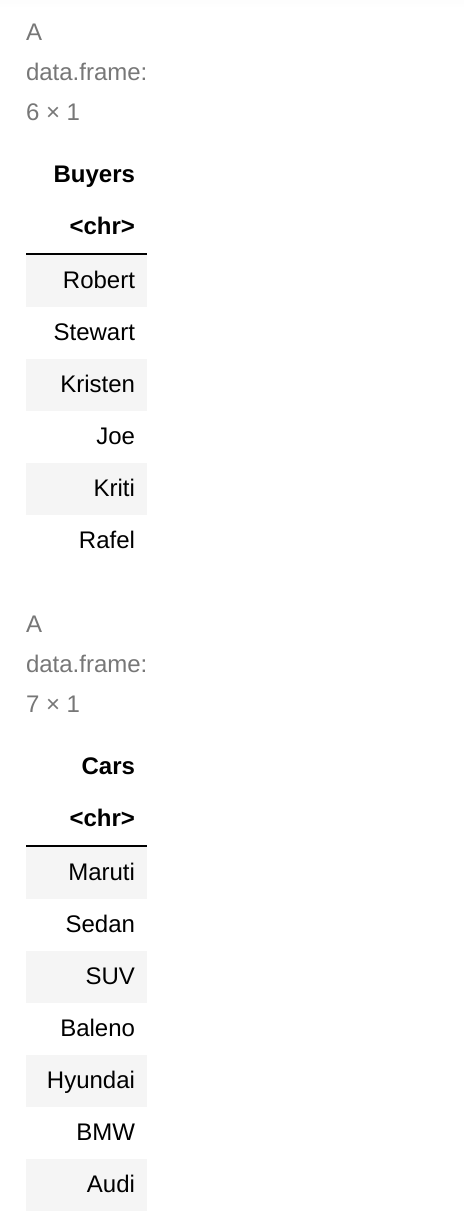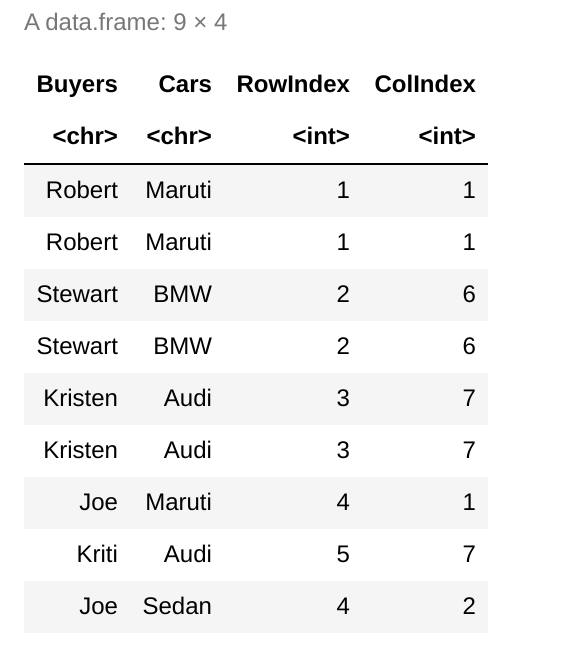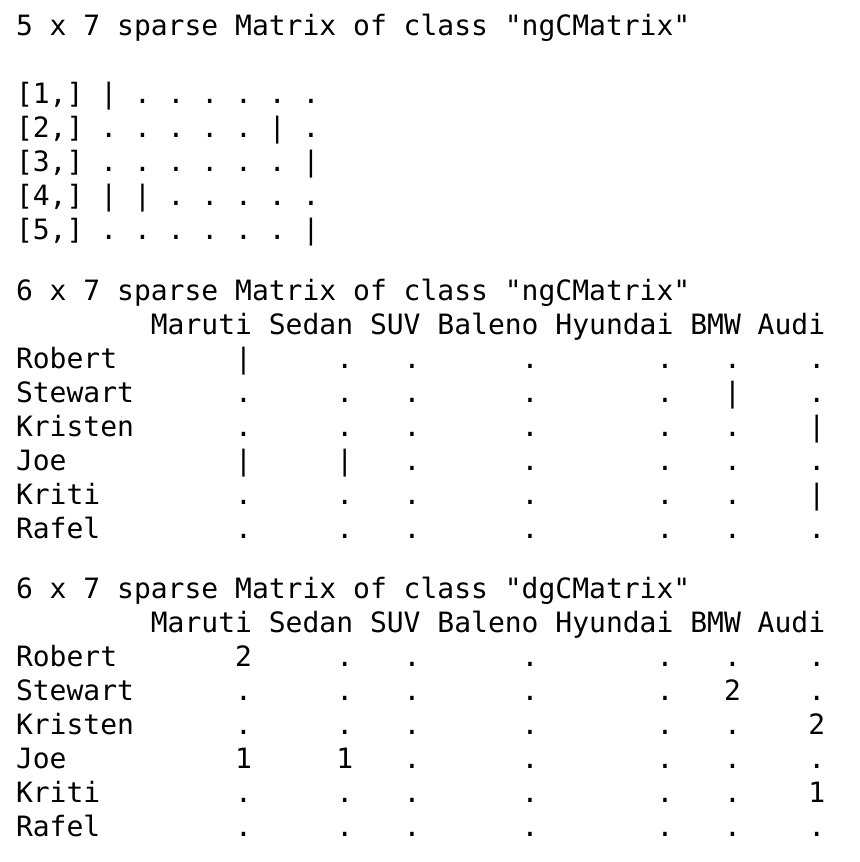# Convert matrix or dataframe to sparse Matrix in R

• Last Updated : 21 Dec, 2021

Sparse matrices are in column-oriented format and they contain mostly null values. The elements in the sparse matrix which are non-null are arranged in ascending order. In this article, we will convert the matrix and dataframe to a sparse matrix in R programming language.

## Converting matrix to sparse matrix

As we know, matrices in R programming language are the objects or collections of elements arranged in a two-dimensional layout. We can construct a matrix in R using the matrix() function.

The first step we are going to do is to install the Matrix package using install.packages(“Matrix”) and then load the package using the library function in R. Next, we are going to construct our matrix using the matrix() function provided by the Matrix package. After the matrix has been generated, create an equivalent sparse matrix using as().

Syntax :

sparsematrix <- as(BaseMatrix, “sparseMatrix”)

Parameters :

• sparsematrix : This is our sample sparse matrix which is to be converted from our base matrix.
• BaseMatrix : This is our sample R matrix.
• “sparseMatrix” : It is the category specified inside the as() function to convert the base R matrix to sparse format.

Example: Converting matrix to sparse matrix in R

## R

 `# loading the Matrix package``library``(Matrix)`` ` `# Constructing a base R matrix ``set.seed``(0)``nrows <- 6L``ncols <- 8L``values <- ``sample``(x = ``c``(0,1,2,3), prob = ``c``(0.6,0.2,0.4,0.8), ``                 ``size = nrows*ncols, replace = ``TRUE``)`` ` `BaseMatrix <- ``matrix``(values, nrow = nrows)``BaseMatrix`` ` `# For converting base matrix to sparse matrix``sparsematrix <- ``as``(BaseMatrix, ``"sparseMatrix"``)``sparsematrix`

Output :## Converting a dataframe to sparse matrix

We know that a dataframe is a table or 2-D array-like structure that has both rows and columns and is the most common way of storing data. We will convert the dataframe to a sparse matrix by using the sparseMatrix() function in R.

Syntax: sparseMatrix(i = ep, j = ep, p, x, dims, dimnames, symmetric = FALSE, triangular = FALSE, index1 = TRUE, repr = “C”, giveCsparse = (repr == “C”), check = “TRUE”, use.last.ij = FALSE)

Parameters :

• i, j : These are the integers of same length that specifies the locations of row and column indices of the matrix.
• p : These are the integer vector of pointers, one for each column or row in the zero-based indexing of rows and columns.
• x : These are the optional values used in matrix entries.
• dims : These are the non-negative integer vectors.
• dimnames : These are the optional lists for ‘dimnames’.
• symmetric : This is the logical variable. If it is specified true, then the resulting matrix should be symmetric and false, otherwise.
• triangular : This is also the logical variable which gives true if the resulting matrix should be triangular and false, otherwise.
• index1 : This is the logical scalar variable. If it is true, then the counting of rows and columns starts at 1. If it is false, then the counting of rows and columns starts at 0.
• repr : These are the character strings which specifies the sparse representation used for result.
• giveCsparse : It is a logical variable indicating whether the resulting matrix is Csparse or Tsparse.
• check : It is a logical variable indicating whether a validity check is performed.
• use.last.ij : It is also logical which indicates in case of duplicate pairs, only the last one should be used.

Example: Converting dataframe to sparse matrix in R

## R

 `library``(Matrix)`` ` `# Creating a table of buyers``buyer <- ``data.frame``(Buyers = ``c``(``"Robert"``, ``"Stewart"``, ``"Kristen"``, ``                               ``"Joe"``, ``"Kriti"``, ``"Rafel"``))``buyer`` ` `# Creating a table of cars``car <- ``data.frame``(Cars = ``c``(``"Maruti"``, ``"Sedan"``, ``"SUV"``, ``"Baleno"``, ``                           ``"Hyundai"``, ``"BMW"``,``"Audi"``))``car`` ` `# Creating a table of orders: (Buyers, cars, units)``# triplets``order <- ``data.frame``(Buyers = ``c``(``"Robert"``, ``"Robert"``, ``"Stewart"``, ``                               ``"Stewart"``, ``"Kristen"``, ``"Kristen"``,``                               ``"Joe"``, ``"Kriti"``, ``"Joe"``),``                    ``Cars = ``c``(``"Maruti"``, ``"Maruti"``, ``"BMW"``, ``"BMW"``, ``                             ``"Audi"``, ``"Audi"``, ``"Maruti"``, ``"Audi"``, ``                             ``"Sedan"``))`` ` `# Insert the RowIndex column, identifying ``# the row index to assign each buyer``order\$RowIndex <- ``match``(order\$Buyers, buyer\$Buyers)`` ` `# Insert the ColIndex column, identifying ``# the column index to assign each car``order\$ColIndex <- ``match``(order\$Cars, car\$Cars)`` ` `# Now inspect``order`` ` `# Creating a basic sparse matrix where element``# (i,j) is true if buyer i bought``# car j and false, otherwise``msparse1 <- ``sparseMatrix``( i = order\$RowIndex, j = order\$ColIndex)``msparse1`` ` `# Creating another sparse matrix to make sure ``# every buyer and every car appears in our matrix``# by setting the dimensions explicitly``msparse2 <- ``sparseMatrix``( i = order\$RowIndex, j = order\$ColIndex, ``                         ``dims = ``c``(``nrow``(buyer), ``nrow``(car)), ``                         ``dimnames = ``list``(buyer\$Buyers, car\$Cars))``msparse2`` ` `# Creating another sparse matrix indicating number ``# of times buyer i bought car j``msparse3 <- ``sparseMatrix``( i = order\$RowIndex, j = order\$ColIndex, x = 1L, ``                         ``dims = ``c``(``nrow``(buyer), ``nrow``(car)),``                         ``dimnames = ``list``(buyer\$Buyers, car\$Cars))``msparse3`

Output :My Personal Notes arrow_drop_up